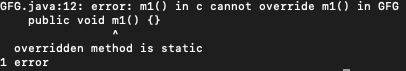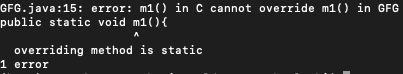• Last Updated : 08 Jun, 2021

Class loading means reading .class file and store corresponding binary data in Method Area. For each .class file, JVM will store corresponding information in Method Area. Now incorporating inheritance in class loading. In java inheritance, JVM will first load and initialize the parent class and then it loads and initialize the child class.

Example

## Java

 `// Java program to Illustrate Class Loading`   `// Importing input output files` `import` `java.io.*;`   `// Helper class 1` `class` `A {`   `    ``// First static block of this class` `    ``static` `    ``{`   `        ``// Print message` `        ``System.out.println(` `            ``"Loading class A 1st static block "``);` `    ``}`   `    ``// Second static block of this class` `    ``static` `    ``{`   `        ``// Print message` `        ``System.out.println(` `            ``"Loading class A 2nd static block B.c="` `            ``+ B.c);` `    ``}`   `    ``// Third static block of this class` `    ``static` `    ``{`   `        ``// Print message` `        ``System.out.println(` `            ``"Loading class A 3rd static block "``);` `    ``}`   `    ``static` `int` `a = ``50``;` `    ``A() {}` `}`   `// Helper class 2 extending from` `// helper class 1` `class` `B ``extends` `A {`   `    ``static` `    ``{` `        ``// Print message` `        ``System.out.println(` `            ``"Loading class B static block A.a="` `+ A.a);` `    ``}`   `    ``static` `int` `c = ``100``;` `}`   `// Main class` `class` `GFG {`   `    ``// Main driver method` `    ``public` `static` `void` `main(String[] args)` `    ``{` `        ``// Execution statement in main class` `        ``new` `B();` `    ``}` `}`

Output

```Loading class A 1st static block

From the above output. it can be said that the parent class loads before the child class and also child class initialize after the parent class. One can check this by executing it with java -verbose Test.

The static block will be executed at the time of class loading, hence at the time of class loading if we want to perform any activity we have to define that inside the static block. In static block inheritance one must remember the following two rules as follows:

1. One can not override a static block as the non-static else compile-time error will be generated.
2. Non-static block can not be overridden as a static block.

Usage of the static block in inheritance is as shown below for the above scenarios as the compiler error that will be generated is shown below for both the cases individually as follows:

Case 1: One can not override a static block as the non-static else compile-time error will be generated

Example

## Java

 `// Java Program to illustrate static blocks in inheritance`   `// Importing all input output classes` `import` `java.io.*;`   `// Class 1- Parent Class` `class` `GFG {`   `    ``// Declaring a static method m1` `    ``public` `static` `void` `m1() {}` `}`   `// Class 2 -  child class of GFG` `class` `c ``extends` `GFG {`   `    ``// Declaring a non- static method m1` `    ``public` `void` `m1() {}` `}`

Output:Case 2: Non-static block can not be overridden as a static block

Example

## Java

 `// Java Program to illustrate static blocks in inheritance`   `// Importing all input output classes` `import` `java.io.*;`   `// Class 1 - Parent class` `class` `GFG {`   `    ``// Declaring a non-static method m1` `    ``public` `void` `m1() {}` `}`   `// Class 2 - child class of parent` `// child class of GFG` `class` `C ``extends` `GFG {`   `    ``// Declaring a static method m1` `    ``public` `static` `void` `m1() {}` `}`

Output:Note: No compile-time error will be generated only and only if both parent and child class methods are static.

My Personal Notes arrow_drop_up
Recommended Articles
Page :